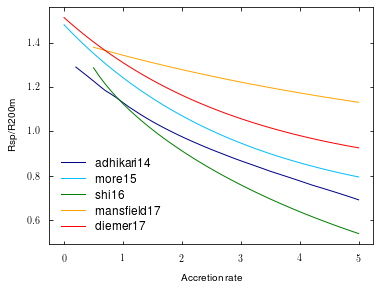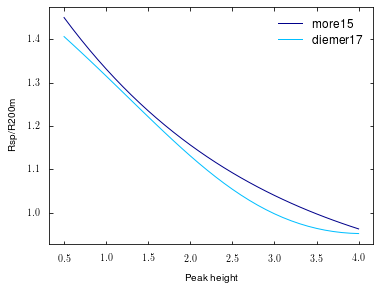# Colossus tutorial: The splashback radius¶

The splashback radius has recently been suggested as a physically motivated definition of the halo boundary. The corresponding Colossus module implements a number of fitting functions for the splashback radius as a function of conventional spherical overdensity definitions, redshift, and mass accretion rate.

In :
from __future__ import print_function
import numpy as np
import matplotlib.pyplot as plt
%matplotlib inline


As always with Colossus, we need to set a cosmology:

In :
from colossus.cosmology import cosmology
cosmology.setCosmology('planck15');


First, let's import the splashback module and print the names of all available splashback models:

In :
from colossus.halo import splashback

for model_name in splashback.models:
print(model_name)

adhikari14
more15
shi16
mansfield17
diemer17


Let's plot the splashback radius predictions of all models as a function of mass accretion rate for a halo with $M_{\rm 200m} = 10^{12} M_{\odot}/h$ at $z = 0$. Not all models may be able to predict $R_{\rm sp}$ at all mass accretion rates, masses, or redshifts, which is why the function returns a mask indicating which input parameters were valid.

In :
from colossus.lss import peaks

cosmology.setCosmology('planck15')
z = 0.0
M200m = 1E12
nu200m = peaks.peakHeight(M200m, z)
Gamma = np.arange(0.0, 5.1, 0.1)

plt.figure()
plt.xlabel('Accretion rate')
plt.ylabel('Rsp/R200m')
for model_name in splashback.models:
RspR200m, mask = splashback.splashbackModel('RspR200m', Gamma = Gamma, nu200m = nu200m, z = z,
model = model_name, rspdef = 'percentile75', statistic = 'median')
plt.plot(Gamma[mask], RspR200m, label = model_name.replace('_', '\_'))
plt.legend();Note that this comparison is not quite accurate because the definitions of mass accretion rate vary between the models. Some models can also predict the splashback mass and radius based only on mass (or peak height). The capabilities of the models are recorded in the models dictionary which we check to filter for models that can handle 'nu200m' as an input quantity:

In :
nu200m = np.arange(0.5, 4.1, 0.1)

plt.figure()
plt.xlabel('Peak height')
plt.ylabel('Rsp/R200m')
for model_name in splashback.models:
if 'nu200m' in splashback.models[model_name].q_in:
RspR200m, mask = splashback.splashbackModel('RspR200m', nu200m = nu200m, z = z,
model = model_name, rspdef = 'percentile75', statistic = 'median')
plt.plot(nu200m[mask], RspR200m, label = model_name.replace('_', '\_'))
plt.legend();For convenience, the splashbackRadius() function provides a wrapper to compute the splashback radius and mass in physical coordinates rather than the ratios provided by the models:

In :
z = 0.0
mdef = 'vir'
Mvir = 1E12
cvir = 10.0

Rsp, Msp, _ = splashback.splashbackRadius(z, mdef, M = Mvir, c = cvir)
print(Rsp, Msp)

288.01847452974846 1202178269899.2158


In this case, we did not tell the function what the mass accretion rate is. This worked because the default model, diemer17, can predict \$R_{\rm sp} as a function of only the halo mass as well. However, the estimate will be much more accurate if we also provide a mass accretion rate:

In :
Rsp, Msp, _ = splashback.splashbackRadius(z, mdef, M = Mvir, c = cvir, Gamma = 3.0)
print(Rsp, Msp)

220.79892707064113 997175166924.9319